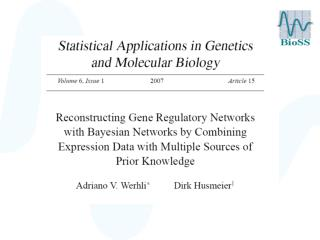DownloadDownload PresentationProblem

Problem

Télécharger la présentationProblem

- - - - - - - - - - - - - - - - - - - - - - - - - - - E N D - - - - - - - - - - - - - - - - - - - - - - - - - - -
Presentation Transcript

1. Problem • Limited number of experimental replications. • Postgenomic data intrinsically noisy. • Poor network reconstruction.

2. Problem • Limited number of experimental replications. • Postgenomic data intrinsically noisy. • Can we improve the network reconstruction by systematically integrating different sources of biological prior knowledge?

3. +

4. + +

5. + + + + …

6. Which sources of prior knowledge are reliable? • How do we trade off the different sources of prior knowledge against each other and against the data?

7. Overview of the talk • Revision: Bayesian networks • Integration of prior knowledge • Empirical evaluation

8. Overview of the talk • Revision: Bayesian networks • Integration of prior knowledge • Empirical evaluation

9. Bayesian networks • Marriage between graph theory and probability theory. • Directed acyclic graph (DAG) representing conditional independence relations. • It is possible to score a network in light of the data: P(D|M), D:data, M: network structure. • We can infer how well a particular network explains the observed data. NODES A B C EDGES D E F

10. Bayesian networks versus causal networks Bayesian networks represent conditional (in)dependence relations - not necessarily causal interactions.

11. Node A unknown A A True causal graph B C B C Bayesian networks versus causal networks

12. Bayesian networks versus causal networks A A A B C B C B C • Equivalence classes: networks with the same scores: P(D|M). • Equivalent networks cannot be distinguished in light of the data. A B C

13. Symmetry breaking A A A B C B C B C A Priorknowledge B C P(M|D) = P(D|M) P(M) / Z D: data. M: network structure

14. P(D|M)

15. P(M) Prior knowledge: B is a transcription factor with binding sites in the upstream regions of A and C

16. P(M|D) ~ P(D|M) P(M)

17. Learning Bayesian networks P(M|D) = P(D|M) P(M) / Z M: Network structure. D: Data

18. Overview of the talk • Revision: Bayesian networks • Integration of prior knowledge • Empirical evaluation

19. Use TF binding motifs in promoter sequences

20. Biological prior knowledge matrix Indicates some knowledge about the relationship between genes i and j Biological Prior Knowledge

21. Biological prior knowledge matrix Indicates some knowledge about the relationship between genes i and j Biological Prior Knowledge Define the energy of a Graph G

22. Notation • Prior knowledge matrix: P  B (for “belief”) • Network structure: G (for “graph”) or M (for “model”) • P: Probabilities

23. Energy of a network Prior distribution over networks

24. Sample networks and hyperparameters • from the posterior distribution • Capture intrinsic inference uncertainty • Learn the trade-off parameters automatically P(M|D) = P(D|M) P(M) / Z

25. Energy of a network Prior distribution over networks

26. Energy of a network Rewriting the energy

27. Approximation of the partition function Partition functionof a perfect gas

28. Multiple sources of prior knowledge

29. MCMC sampling scheme

30. Sample networks and hyperparameters from the posterior distribution Proposal probabilities Metropolis-Hastings scheme

31. Bayesian networkswith biological prior knowledge • Biological prior knowledge: Information about the interactions between the nodes. • We use two distinct sources of biological prior knowledge. • Each source of biological prior knowledge is associated with its own trade-off parameter:b1 and b2. • The trade off parameter indicates how much biological prior information is used. • The trade-off parameters are inferred. They are not set by the user!

32. Bayesian networkswith two sources of prior Source 2 Source 1 Data BNs + MCMC b1 b2 Recovered Networks and trade off parameters

33. Bayesian networkswith two sources of prior Source 2 Source 1 Data BNs + MCMC b1 b2 Recovered Networks and trade off parameters

34. Bayesian networkswith two sources of prior Source 2 Source 1 Data BNs + MCMC b1 b2 Recovered Networks and trade off parameters

35. Overview of the talk • Revision: Bayesian networks • Integration of prior knowledge • Empirical evaluation

36. Evaluation • Can the method automatically evaluate how useful the different sources of prior knowledge are? • Do we get an improvement in the regulatory network reconstruction? • Is this improvement optimal?

37. Raf regulatory network From Sachs et al Science 2005

38. Raf regulatory network

39. Evaluation: Raf signalling pathway • Cellular signalling network of 11 phosphorylated proteins and phospholipids in human immune systems cell • Deregulation  carcinogenesis • Extensively studied in the literature  gold standard network

40. DataPrior knowledge

41. Flow cytometry data • Intracellular multicolour flow cytometry experiments: concentrations of 11 proteins • 5400 cells have been measured under 9 different cellular conditions (cues) • Downsampling to 100 instances (5 separate subsets): indicative of microarray experiments

42. Microarray example Spellman et al (1998) Cell cycle 73 samples Tu et al (2005) Metabolic cycle 36 samples time time Genes Genes

43. DataPrior knowledge